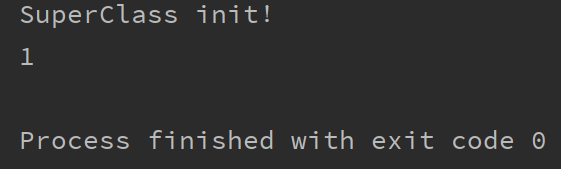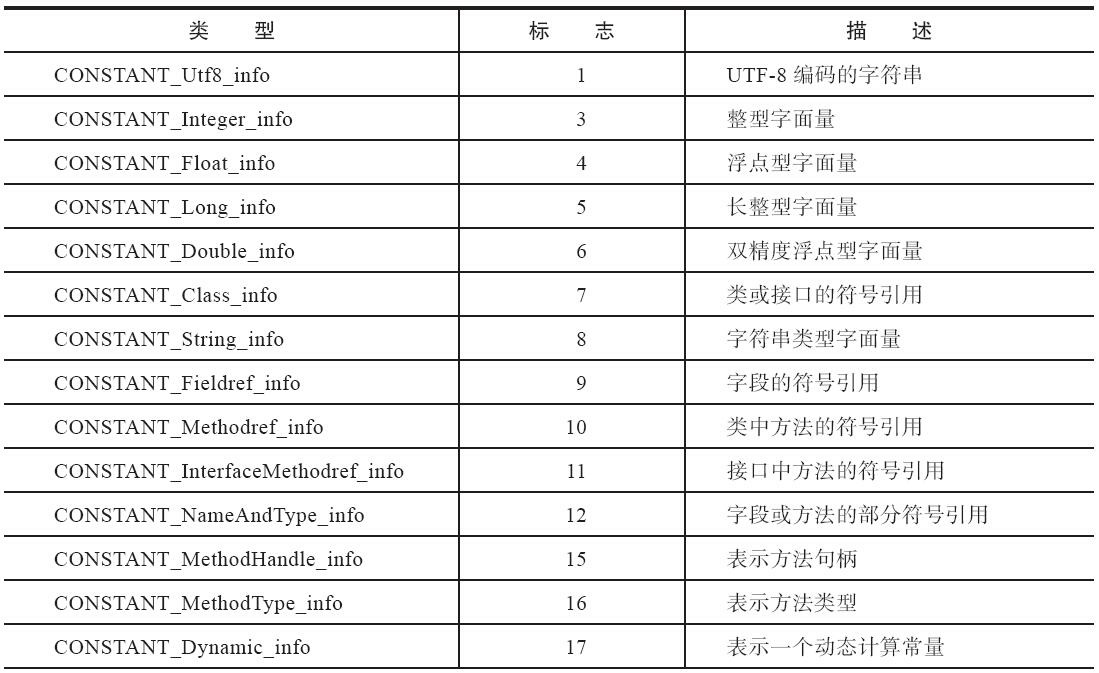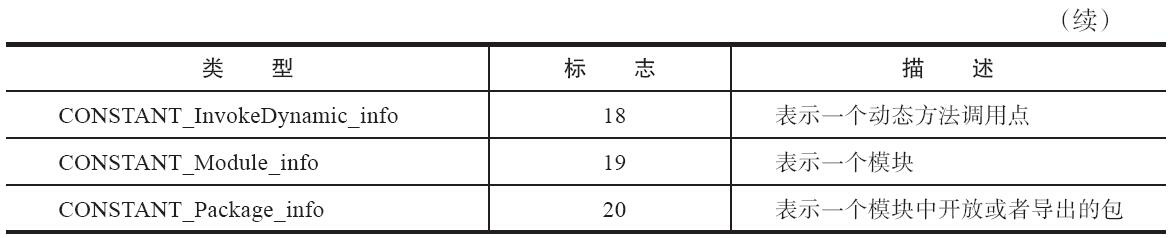This website requires JavaScript.

# 《深入理解 Java 虚拟机》中一题引发的思考

### 2 - 类初始化时机

#### 2.1 - 原题

``````public class SuperClass {
static {
System.out.println("SuperClass init！");
}

public static int VALUE = 1;
}

public class SubClass extends SuperClass {
static {
System.out.println("SubClass init！");
}
}

public class Main {

public static void main(String[] args) {
System.out.println(SubClass.VALUE);
}
}
``````

#### 2.2 - 变式一

``````public class SuperClass {
static {
System.out.println("SuperClass init");
}

// public static int VALUE = 1;
public final static int VALUE = 1; // 添加一个 final 修饰
}
``````

``````// Main.class
public class Main {
public Main() {
}

public static void main(String[] args) {
System.out.println(1);
}
}
``````

`Main`类在编译的时候直接把 `SubClass.VALUE` 优化成了值"1"。这和一开始的猜测还是有些出入，`Main`类不是被 JVM 在运行时优化的，而是在编译器就直接被优化了。

#### 2.3 - 变式二

``````public class SuperClass {
static {
System.out.println("SuperClass init！");
}

// public static int VALUE = 1;
// public final static int VALUE = 1;
public final static Integer VALUE = 1; // 把 VALUE 改成 Integer 包装类
}
``````#### 2.4 - 变式三

``````public class SuperClass {
static {
System.out.println("SuperClass init！");
}

// public static int VALUE = 1;
// public final static int VALUE = 1;
// public final static Integer VALUE = 1;
public final static String VALUE = "1"; // 把 VALUE 改成 String
}
``````

``````// Main.class
public class Main {
public Main() {
}

public static void main(String[] args) {
System.out.println("1");
}
}
``````

### 3 - 编译器优化技术---条件常量传播

#### 3.1 - 优化常量

``````public class Main {
public static final int a = 1; // 全局静态常量

public static void main(String[] args) {
final int b = 2; // 局部常量
System.out.println(a);
System.out.println(b);
}
}
``````

``````// Main.class
public class Main {
public static final int a = 1;

public static void main(String[] args) {
int b = true;
System.out.println(1);
System.out.println(2);
}
}
``````

#### 3.2 - 优化常量表达式

``````public class Main {
public static void main(String[] args) {
final int a = 3 * 4 + 5 - 6;
int b = 10;
if (a > b) {
System.out.println(a);
}
}
}
``````

``````// Main.class
public class Main {
public static void main(String[] args) {
int a = true;
int b = 10;
if (11 > b) {
System.out.println(11);
}

}
}
``````

#### 3.3 - 优化字符串拼接

``````public class Main {
public static void main(String[] args) {
final String str = "hel" + "lo";
System.out.println(str);
System.out.println("hello" == str);
}
}
``````

``````// Main.class
public class Main {
public static void main(String[] args) {
String str = "hello";
System.out.println("hello");
System.out.println(true);
}
}
``````

#### 3.4 - 编译器条件常量传播带来的风险

##### 3.4.1 - 常量反射失效

``````public class Main {
public static final String VALUE = "A";

public static void main(String[] args) throws NoSuchFieldException, IllegalAccessException {
Class<Main> mainClass = Main.class;
Field value = mainClass.getField("VALUE");
value.setAccessible(true);

// 去除 A 的 final 修饰符
Field modifiersField = Field.class.getDeclaredField("modifiers");
modifiersField.setAccessible(true);
modifiersField.setInt(value, value.getModifiers() & ~Modifier.FINAL);

value.set(null, "B");
System.out.println(VALUE); // 实际还是输出 "A"
}
}
``````

##### 3.4.2 - 部分编译

``````// Constant.java
public class Constant {
public final static String VALUE = "A";
}

// Main.java
public class Main {
public static void main(String[] args) {
System.out.println(Constant.VALUE);
}
}
``````

### 4 - 常量、静态常量池、动态常量池

#### 4.2 - 静态常量池

（静态）常量池可以比喻为 Class 文件里的资源仓库，它是 Class 文件结构中与其他项目关联最多的数据，通常也是占用 Class 文件空间最大的数据项目之一，另外，它还是在 Class 文件中第一个出现的表类型数据项目。

（静态）常量池里面存储的数据项目类型如下表：1

``````public class Main {
final static String A = "A";
final static int B = 1;
final static Integer C = 2;

public static void main(String[] args) {
System.out.println(A);
System.out.println(B);
System.out.println(C);
}
}
``````

### 5 - 结语

《深入理解 Java 虚拟机》确实是一本很好的书，反复读过几次，每次都有新的收获，这次更是由于自己在打源码的时候的"不小心失误"有了意料之外的收获。

### 参考

0条评论